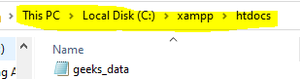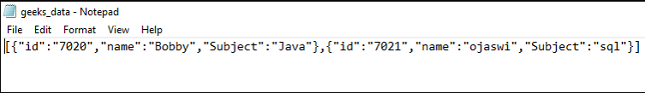# How to generate Json File in PHP ?

• Last Updated : 21 May, 2021

In this article, we are going to generate a JSON file in PHP by using an array. JSON stands for JavaScript object notation, which is used for storing and exchanging data. JSON is text, written with JavaScript object notation.

Structure:

```{"data":[
{ "sub_data1":"value1", "sub_data2":"value2","sub_data_n":"value n" },
{ "sub_data2":"value2","sub_data2":"value2", "sub_data_n":"value n" },
{ "sub_data n":"value n ", "sub_data2":"value2","sub_data_n":"value n" }
]}```

Example:

```[{"id":"7020","name":"Bobby","Subject":"Java"},
{"id":"7021","name":"ojaswi","Subject":"sql"}]```

Properties:

1. JSON doesn’t use an end tag
2. It is shorter.
3. It is quicker to read and write.
4. It can use arrays.

Approach: In this article, we can generate JSON data using an array., create an array

Syntax:

```\$array = Array (
"number" => Array (
"data1" => "value1",
"data2" => "value2",
"data n" => "valuen"
),
"number" => Array (
"data1" => "value1",
"data2" => "value2",
"data n" => "valuen"
)
);```

Example:

```\$array = Array (
"0" => Array (
"id" => "7020",
"name" => "Bobby",
"Subject" => "Java"
),
"1" => Array (
"id" => "7021",
"name" => "ojaswi",
"Subject" => "sql"
)
);```

Use json_encode() to convert array to JSON. It is used to convert array to JSON

Syntax:

`json_encode(array_input);`

Example: Place the file in the path using file_put_contents()

`\$json = json_encode(\$array);`

The file_name is the JSON to be saved and json_object is the object after JSON from the array is created.

Syntax:

`file_put_contents(file_name.json.json_object);`

Example:

`file_put_contents("geeks_data.json", \$json);`

PHP code:

## PHP

 ` Array ( ` `        ``"id"` `=> ``"7020"``, ` `        ``"name"` `=> ``"Bobby"``, ` `        ``"Subject"` `=> ``"Java"` `    ``), ` `    ``"1"` `=> Array ( ` `         ``"id"` `=> ``"7021"``, ` `        ``"name"` `=> ``"ojaswi"``, ` `        ``"Subject"` `=> ``"sql"` `    ``) ` `); ` ` `  `// encode array to json ` `\$json` `= json_encode(``\$array``); ` `//display it  ` `echo` `"\$json"``; ` `//generate json file ` `file_put_contents``(``"geeks_data.json"``, ``\$json``); ` ` `  `?>`

Output:

```[{"id":"7020","name":"Bobby","Subject":"Java"},
{"id":"7021","name":"ojaswi","Subject":"sql"}]```
• The JSON file is created in your path.• The data present in the created filegeeks_data

My Personal Notes arrow_drop_up
Recommended Articles
Page :